Courses

# Chapter 2 Cathode Ray Oscilloscope - Notes, Electrical Measurement, Electrical Engineering Electrical Engineering (EE) Notes | EduRev

## Electrical Engineering (EE) : Chapter 2 Cathode Ray Oscilloscope - Notes, Electrical Measurement, Electrical Engineering Electrical Engineering (EE) Notes | EduRev

The document Chapter 2 Cathode Ray Oscilloscope - Notes, Electrical Measurement, Electrical Engineering Electrical Engineering (EE) Notes | EduRev is a part of the Electrical Engineering (EE) Course Electrical Engineering SSC JE (Technical).
All you need of Electrical Engineering (EE) at this link: Electrical Engineering (EE)

Introduction :

• The CRO is a device that allows the amplitude of electrical signals, whether they be voltages, current, power etc. to be displayed primarily as a function of time.
• The cathode ray oscilloscope (CRO) is a very useful and versatile laboratory instrument used for display, measurement and analysis of waveforms and other phenomena in electrical and electronics circuits.

Cathode Ray Tube (CRT) :

• The “Heart of the CRO” is the CRT, which generates the electron beam, accelerates the beam to a high velocity, deflects the beam to create the image and contains the phosphor screen where the electron beam eventually becomes visible.
•  The main parts of a CRTs are following :
• Electron gun assembly
• Deflection plate assembly
• Fluorescent screen
• Glass envelope
• Base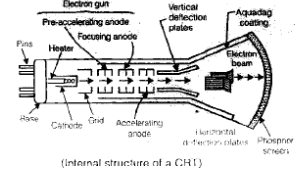Electron gun

• The source of focused and accelerated electron beam is the electron gun.
• The electron gun which emits electrons and forms them into a beam consists of several elements which are given below.

Heating element

• Heating element used to heat up the cathode.

Cathode

• It is cylindrical shaped with layer of barium and strontium oxide is deposited on the end of the cathode which is to obtain high emission of electrons at moderate temperatures.

Control grid

• The control grid is used to control the number of electron emitted from cathode.
• This control grid is usually a nickel cylinder, with a centrally located hole, coaxial with the CRT axis.
• The grid with its negative bias controls the number of electrons emitted from the cathode and hence the intensity of electron beam is controlled by the grid.

Pre-accelerating and accelerating anode:

• The electrons, emitted from the cathode and passing through the hole in the control grid are accelerated by the high positive potential which is applied to the pre-accelerating and accelerating anode.
• The pre-accelerating anode and the accelerating anode are connected to a common positive high voltage of 1500 V.

Focusing anode

• The electron beam is focusing an electron beam.
(i) Electrostatic focusing
(ii) Electromagnetic focusing

Deflecting plates

• From figure we observe that the electron beam, after leaving the electron gun passes through two pairs of deflection plates.

Vertical deflecting plates or Y-plates

• It is responsible for vertical deflection of electron beam.
• These plates are supplied with external signal fed via one of the input channel of CRO.

Horizontal deflecting plates or X-plates:

• These plates are responsible for horizontal motion of electron beam.
•  In normal mode of CRO, horizontal plates are connected to output signal of sweep generator which is inside the CRO.
• For the display of a waveform, horizontal plates are given a saw tooth wave which results into continuous motion electron beam from left to right on the screen.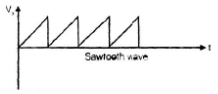## Application of CRO :

Measurement of voltages and Currents

• The electrostatic deflection given in equation shows that the deflection is proportional to the deflection-plate voltage. The value of a current can be obtained by measuring the voltage drop across a known resistance connected in the circuit.
• Direct voltages may be obtained from the static deflection of the spot, alternating voltages from the length of the line produced when the voltage is applied to Y-plates while no voltage is applied to X-plates. The length of this line corresponds to peak to peak voltage.
• When dealing with sinusoidal voltages, the rms value is given by dividing the peak to peak voltage by 2 √2.

Measurement of Phase

• It is interesting to consider the characteristics of pattern that appear on the screen of a CRT when sinusoidal voltages are simultaneously applied to horizontal and vertical deflection plates. These patterns are called “Lissajous patterns”.

Measurement of Frequency :

• Lissajous pattern may be used for accurate measurement of frequency.
• Frequency of signal with CRO can be determined by operation it in X-Y mode. In this Y-input channel and a signal of known frequency is connected to X-input channel.

The frequency of the signal can be given by using following formula,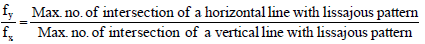where,
fy = unknown frequency
fx = known frequency

also,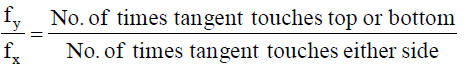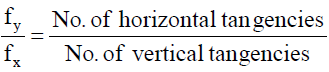where, fy = frequency of signal applied to Y-plates
fx = frequency of signal applied to X-plates

Offer running on EduRev: Apply code STAYHOME200 to get INR 200 off on our premium plan EduRev Infinity!

65 docs|37 tests

,

,

,

,

,

,

,

,

,

,

,

,

,

,

,

,

,

,

,

,

,

,

,

,

,

,

,

;